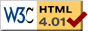### Journée-séminaire de combinatoire

#### (équipe CALIN du LIPN, université Paris-Nord, Villetaneuse)

Le 03 mars 2009 à 14h en B107, Alin Bostan nous parlera de : The full counting function of Gessel walks is algebraic

Résumé : The aim of the talk is to show how a difficult combinatorial problem has been recently solved using an experimental-mathematics approach combined with (rather involved) computer algebra techniques. More precisely, let $f(n,i,j)$ denote the number of lattice walks in the quarter plane which start at the origin, end at the point $(i,j)$, and consist of $n$ unit steps going either west, south-west, east, or north-east. In the early nineties, Ira Gessel conjectured that the sequence of excursions $f(n,0,0)$ is holonomic. We will present the computer-driven discovery and proof of the following generalization, obtained in August 2008 together with Manuel Kauers: the full generating series $F(t,x,y) = \displaystyle{\sum_{i,j,n \geq 0} f(n,i,j) x^i y^j t^n}$ is an algebraic function.

 Dernière modification : Monday 24 January 2022Contact pour cette page : Cyril.Banderier at lipn.univ-paris13.fr